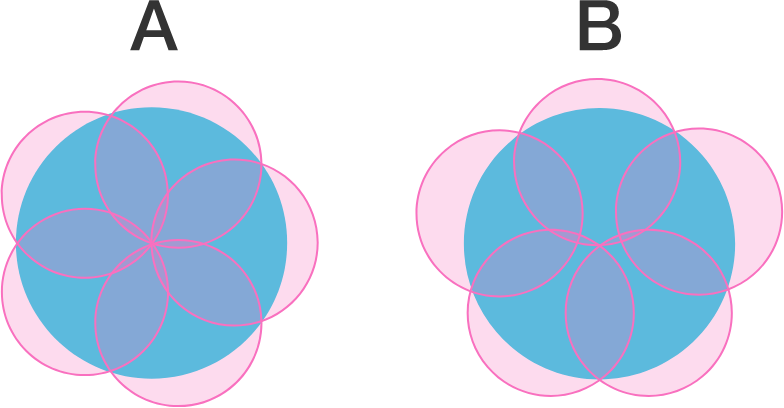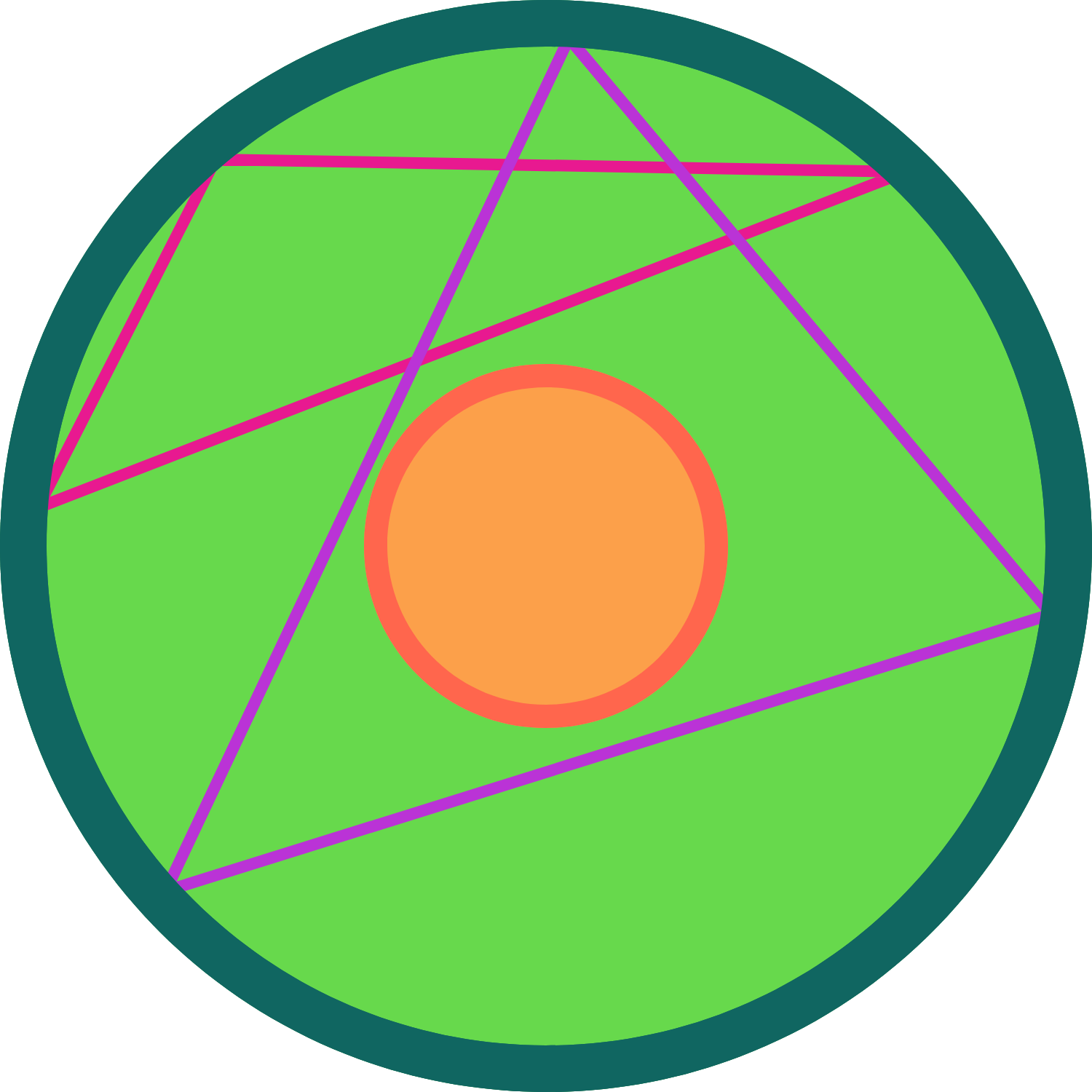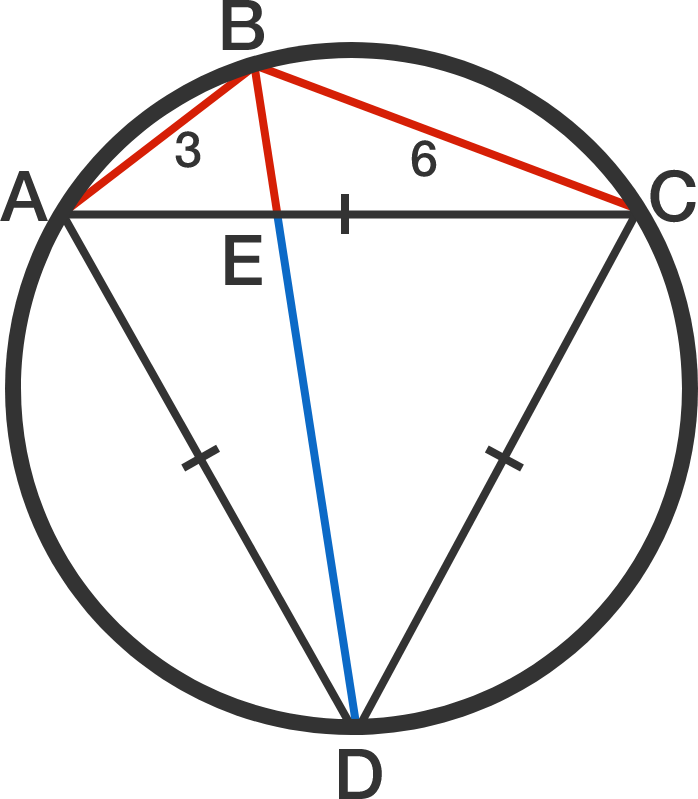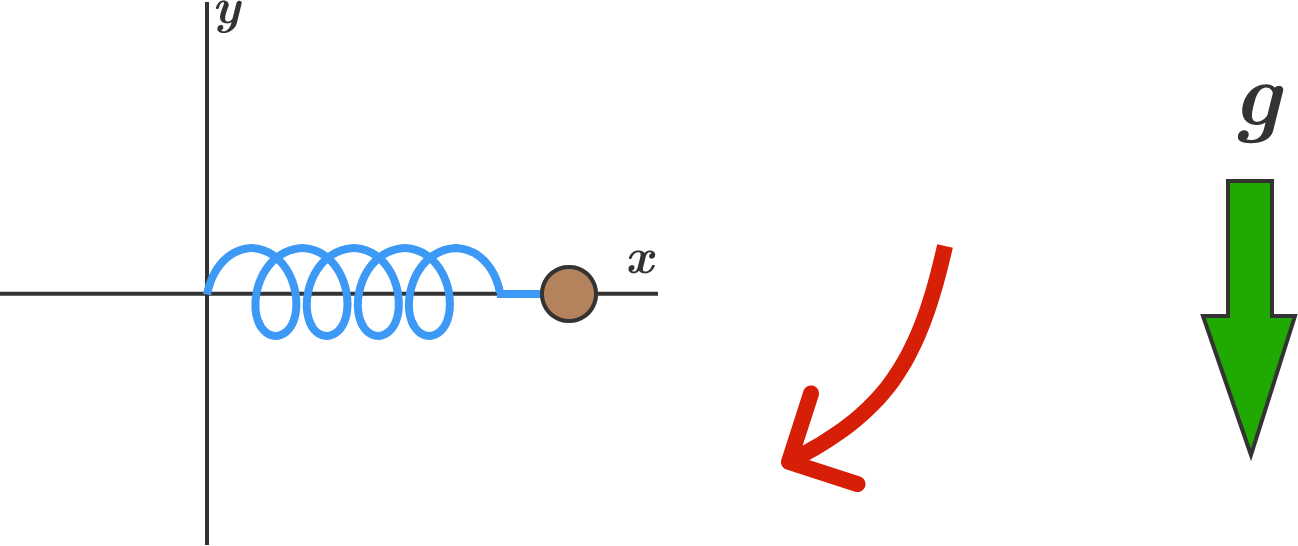# Problems of the Week

Contribute a problem

# 2017-05-15 AdvancedYou go to a carnival and decide to play the Cover the Spot game.

The rules are simple: you must cover the largest circular spot possible using 5 identical circular disks. Which configuration can cover a larger spot?

A) All 5 disks pass through the center of the spot.
B) Exactly 3 disks pass through the center of the spot.Circles $$L$$ and $$l$$ share the same center. Circle $$L$$ has radius $$3r$$ and circle $$l$$ has radius $$r.$$ Points $$A, B, C$$ are chosen on the circumference of circle $$L$$ uniformly at random.

What is the probability that the centroid of $$\triangle ABC$$ lies in the interior of circle $$l$$?A cyclic quadrilateral $$ABCD$$ is constructed within a circle such that $$AB = 3, BC = 6,$$ and $$\triangle ACD$$ is equilateral, as shown to the right.

If $$E$$ is the intersection point of both diagonals of $$ABCD$$, what is the length of $$ED,$$ the blue line segment in the diagram?

$\frac{m^3-n^3}{m^2+n^2-mn}$

Find the sum of all prime numbers less than 900 that can be expressed as the above fraction where $$m$$ and $$n$$ are positive integers.In the $$xy$$-coordinate system, a $$\SI{1}{\kilo\gram}$$ mass is attached to one end of a massless ideal spring. The other end of the spring is fixed at the origin. The spring constant is $$k = \SI[per-mode=symbol]{10}{\newton\per\meter}$$, and the spring's unstretched length is $$\SI{1}{\meter}$$.

The mass is initially being held in the air horizontally at $$(x,y) = (\SI{1}{\meter}, \SI{0}{\meter}),$$ and then is released so that gravity pulls it downwards. How far is the mass from the origin right at the moment it first crosses the (vertical) $$y$$-axis?


Details and Assumptions:

• Give your answer in meters, to 2 decimal places.
• There is an ambient downward gravitational acceleration of $$\SI[per-mode=symbol]{10}{\meter\per\second\squared}$$.
• For the sake of this problem, assume that the spring can be stretched to great lengths.
×

Problem Loading...

Note Loading...

Set Loading...